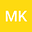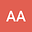Nonexistence of Global Solutions of Systems of Time Fractional Differential equations posed on the Heisenberg group
••alrazi abdeljabbar
Khalifa University of Science and Technology
Author Profile## Abstract

We first consider the nonlinear time fractional diffusion equation D^{1+α}u+D^β u−∆_{H} u=|u|^p posed on the Heisenberg group H, where 1 < p is a positive real nimber to be specified later; D^δ_{0|t} is the Liouville-Caputo derivative of order δ. For 0 < α < 1,0 < β ≤ 1. This equation interpolates the heat equation and the wave equation with the linear damping D^β_{0|t}u. We present the Fujita exponent for blow-up. Then establish sufficient conditions ensuring non-existence of local solutions. We extend the analysis to the case of the system D^{1+α}u+D^β u−∆_{H} u=|v|^q D^{1+δ}v+D^γ v−∆_{H} v=|u|^p. Our method of proof is based on the nonlinear capacity method.
14 Nov 2021Submitted to Mathematical Methods in the Applied Sciences
15 Nov 2021Submission Checks Completed
15 Nov 2021Assigned to Editor
19 Nov 2021Reviewer(s) Assigned
05 Jan 2022Review(s) Completed, Editorial Evaluation Pending
06 Jan 2022Editorial Decision: Revise Minor